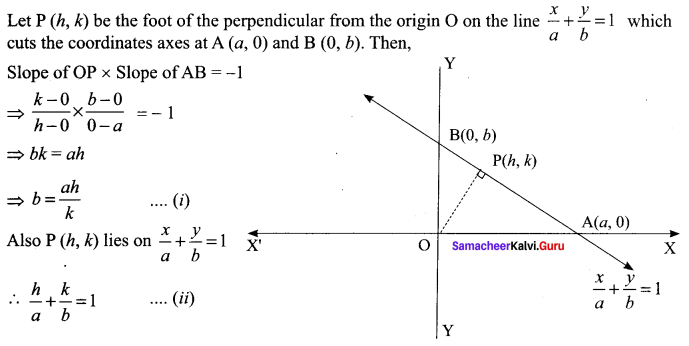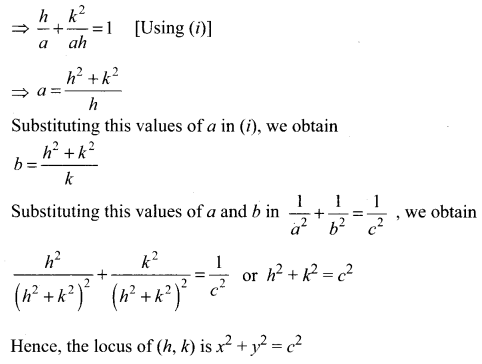## Tamilnadu Samacheer Kalvi 11th Maths Solutions Chapter 6 Two Dimensional Analytical Geometry Ex 6.1

Question 1.
Find the locus of P, if for all values of a, the co-ordinates of a moving point P is
(i) (9 cos α, 9 sin α)
(ii) (9 cos α, 6 sin α)
Solution:
(i) Let P(h, k) be the moving point.
We are given h = 9 cos α and k = 9 sin α and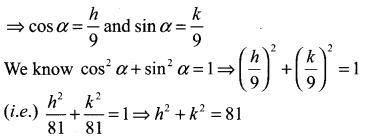∴ locus of the point is x2 + y2 = 81

(ii) Let P(h , k) be a moving point.
We are given h = 9 cos α and k = 6 sin α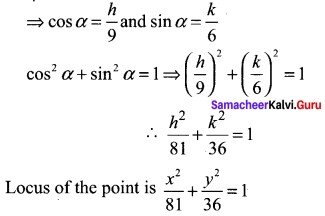Question 2.
Find the locus of a point P that moves at a constant distant of
(i) Two units from the x-axis
(ii) Three units from the y-axis.
Solution:
(i) Let the point (x, y) be the moving point. Equation of a line at a distance of 2 units from x-axis is k = 2
So the locus is y = 2
(i.e.) y – 2 = 0
435

(ii) Equation of a line at a distance of 3 units from y-axis is h = 3
So the locus is x = 3 (i.e.) x – 3 = 0Question 3.
If θ is a parameter, find the equation of the locus of a moving point, whose coordinates are x – a cos3 θ, y = a sin3 θ
Solution: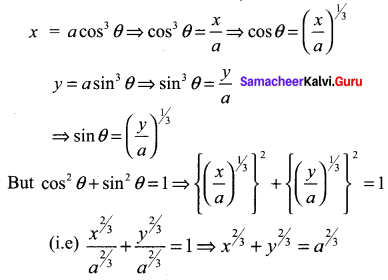Question 4.
Find the value of k and b, if the points P (-3, 1) and Q (2, b) lie on the locus of x2 – 5x + ky = 0.
Solution:
Given P (-3, 1) lie on x2 – 5x + ky = 0
⇒ (-3)2 – 5(-3) + k(1) = 0
9 + 15 + k = 0 ⇒ k = -24
Q (2, b) lie on x2 – 5x + ky = 0
(2)2 – 5(2) + k(b) = 0 ⇒ 4 – 5(2) – 24b = 0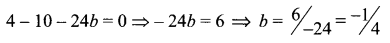Question 5.
A straight rod of length 8 units slides with its ends A and B always on the x and y-axis respectively. Find the locus of the mid point of the line segment AB.
Solution:
Let P (h, k) be the moving point A (a, 0) and B (0, b) P is the mid point of AB.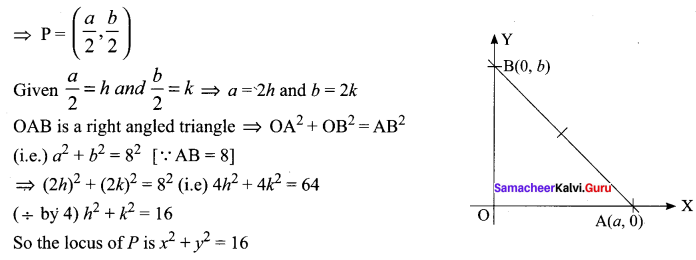Question 6.
Find the equation of the locus of a point such that the sum of the squares of the distance from the points (3, 5), (1, -1) is equal to 20.
Solution:
Let P (h, k) be the moving point
Let the given point be A (3, 5) and B (1, -1)
We are given PA2 + PB2 = 20
⇒ (h – 3)2 + (k – 5)2 + (h – 1)2 + (k + 1)2 = 20
⇒ h2 – 6h + 9 + k2 – 10k + 25 + h2 – 2h + 1 + k2 + 2k + 1 = 20
(i.e.) 2h2 + 2k2 – 8h – 8k + 36 – 20 = 0
2h2 + 2k2 – 8h – 8k + 16 = 0
(÷ by 2 ) h2 + k2 – 4h – 4k + 8 = 0
So the locus of P is x2 + y2 – 4x – 4y + 8 = 0Question 7.
Find the equation of the locus of the point P such that the line segment AB, joining the points A (1, -6) and B (4, -2), subtends a right angle at P.
Solution:
Let P (h, k) be the moving point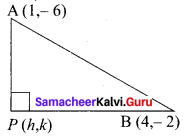Given A (1, – 6) and B (4, – 2),
Since ∆APB = 90°, PA2 + PB2 = AB2
(i.e.) (h – 1)2 + (k + 6)2 + (h – 4)2 + (k + 2)2 = (4 – 1)2 + (-2 + 6)2
(i.e) h2 + 1 – 2h + k2 + 36 + 12k + h2 + 16 – 8h + k2 + 4 + 4k = 32 + 42 = 25
2h2 + 2k2 -10h + 16k + 57 – 25 = 0
2h2 + 2k2 – 10h + 16k + 32 = 0
(÷ by 2)h2 + k2 – 5h + 8k + 16 = 0
So the locus of P is x2 + y2 – 5x + 8y + 16 = 0

Question 8.
If O is origin and R is a variable point on y2 = 4x, then find the equation of the locus of the mid-point of the line segment OR.
Solution:
Let P(h, k) be the moving point
We are given O (0, 0). Let R = (a, b)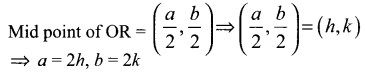Substituting a, b values is y2 = 4x
we get (2k)2 = 4 (2h)
(i.e) 4k2 = 8h
(÷ by 4) k2 = 2h
So the locus of P is y2 = 2x

Question 9.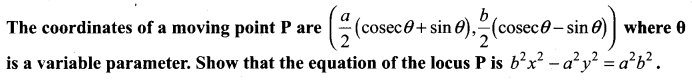Solution: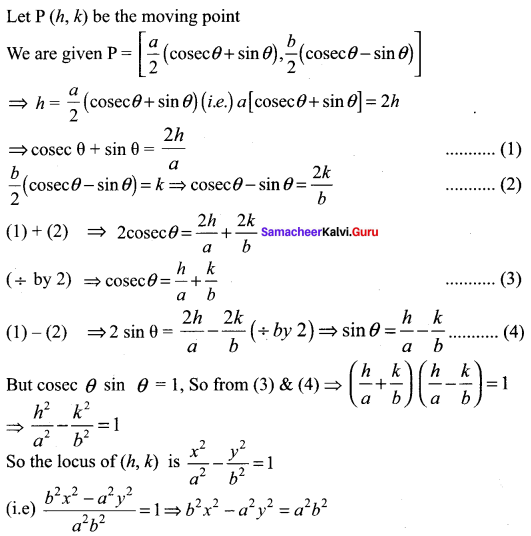Question 10.
If P (2, -7) is a given point and Q is a point on 2x2 + 9y2 = 18, then find the equations of the locus of the mid-point of PQ.
Solution:
P = (2, -7); Let (h, k) be the moving point Q = (a, b)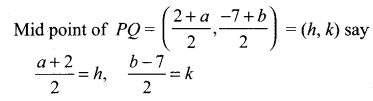⇒ a = 2h – 2,
b = 2k + l
Q is a point on 2x2 + 9y2 = 18 (i.e) (a, b) is on 2x2 + 9y2 = 18
⇒ 2(2h – 2)2 + 9 (2k + 7)2 = 18
(i.e) 2 [4h2 + 4 – 8h] + 9 [4k2 + 49 + 28k] – 18 = 0
(i.e) 8h2 + 8 – 16h + 36k2 + 441 + 252k – 18 = 0
8h2 + 36k2 – 16h + 252k + 431 = 0
The locus is 8x2 + 36y2 – 16x + 252y + 431 = 0Question 11.
If R is any point on the x-axis and Q is any point on the y-axis and Pis a variable point on RQ with RP = b, PQ = a. then find the equation of locus of P.
Solution:
P = (x, 0), Q = (0, y), R (h, k) be a point on RQ such that PR : RQ = b : a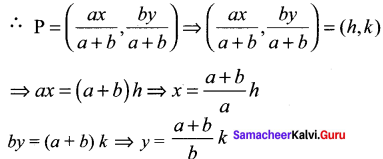From the right angled triangle OQR, OR2 + OQ2 = QR2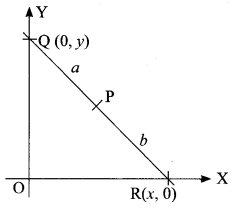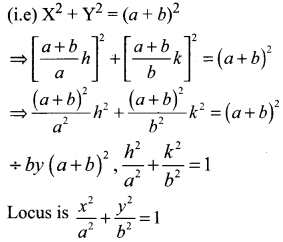Question 12.
If the points P (6, 2) and Q (-2, 1) and R are the vertices of a ∆PQR and R is the point on the locus y = x2 – 3x + 4, then find the equation of the locus of centroid of ∆PQR.
Solution:
P (6, 2), Q (-2, 1). Let R = (a, b) be a point on y = x2 – 3x + 4.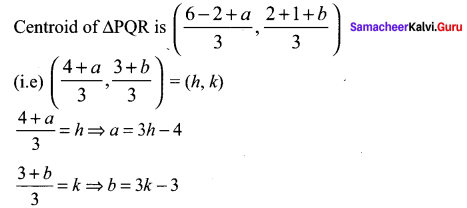But (a, b) is a point on y = x2 – 3x + 4
b = a2 – 3a + 4
(i.e) 3k – 3 = (3h – 4)2 – 3(3h – 4) + 4
(i.e) 3k – 3 = 9h2 + 16 – 24h – 9h + 12 + 4
⇒ 9h2 – 24h – 9h + 32 – 3k + 3 = 0
(i.e) 9h2 – 33h – 3k + 35 = 0,
Locus of (h, k) is 9x2 – 33x – 3y + 35 = 0

Question 13.
If Q is a point on the locus of x2 + y2 + 4x – 3y + 7 = 0 then find the equation of locus of P which divides segment OQ externally in the ratio 3 : 4, where O is origin.
Solution:
Let (h, k) be the moving point O = (0, 0);
Let PQ = (a, b) on x2 + y2 + 4x – 3y + 7 = 0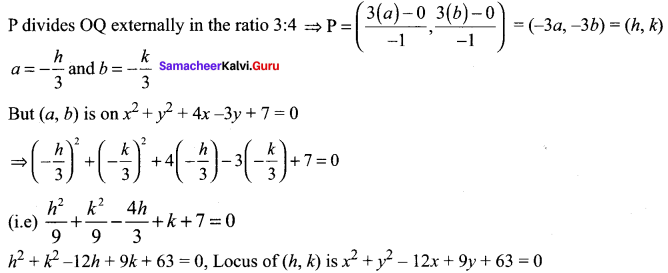Question 14.
Find the points on the locus of points that are 3 units from x – axis and 5 units from the point (5, 1).
Solution:
A line parallel to x-axis is of the form y = k. Here k = 3 ⇒y = 3
A point on this line is taken as P (a, 3). The distance of P (a, 3) from (5, 1) is given as 5 units
⇒ (a – 5)2 + (3 – 1)2 = 52
a2 + 25 – 10a + 9 + 1 – 6 = 25
a2 – 10a + 25 + 4 – 25 = 0
a2 – 10a + 4 = 0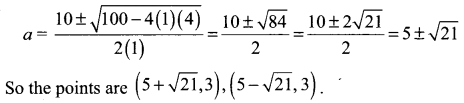Question 15.
The sum of the distance of a moving point from the points (4, 0) and (-4, 0) is always 10 units. Find the equation of the locus of the moving point.
Solution:
Let P (h, k) be a moving point
Here A = (4, 0) and B = (-4, 0)
Given PA + PB = 10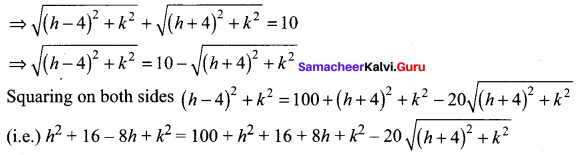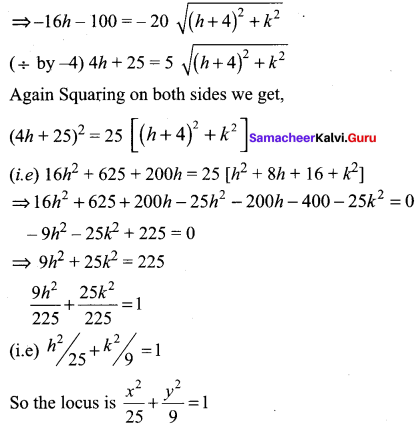### Samacheer Kalvi 11th Maths Solutions Chapter 6 Two Dimensional Analytical Geometry Ex 6.1 Additional Questions

Question 1.
If the sum of the distance of a moving point in a plane from the axis is 1, then find the locus of the point.
Solution:
Let coordinates of a moving point P be (x, y).
Given that the sum of the distances from the axis to the point is always 1.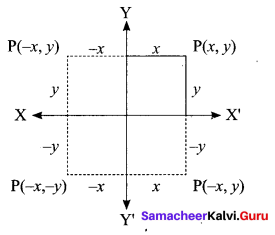∴ |x| + |y| = 1 ⇒ x + y = 1
⇒ -x – y = 1 ⇒ x – y = 1
Hence, these equations give us the locus of the point P which is a square.

Question 2.
A point moves so that square of its distance from the point (3, -2) is numerically equal to its distance from the line 5x -12y = 3. The equation of its locus is ……..
Solution:
The given equation of line is 5x – 12y = 3 and the given point is (3, -2).
Let (a, b) be any moving point.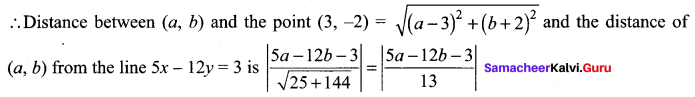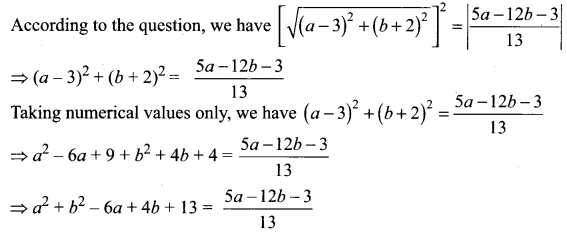⇒ 13a2 + 13b2 – 78a + 52b + 169 = 5a – 12b – 3
⇒ 13a2 + 13b2 – 83a + 64b + 172 = 0
So, the locus of the point is 13x2 + 13y2 – 83x + 64y + 172 = 0Question 3.
Find the Locus of the mid points of the portion of the line x cos θ + y sin θ = p intercepted between the axis.
Solution:
Given equation of the line is x cos θ + y sin θ = p … (i)
Let C (h, k) be the mid point of the given line AB where it meets the two axis at A (a, 0) and B (0, b).
Since (a, 0) lies on eq (i) then “a cos θ + θ = p”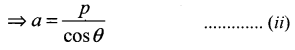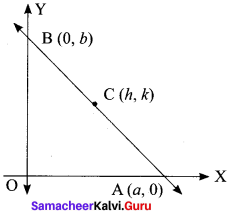B (0, b) also lies on the eq (i) then 0 + b sin θ = p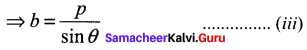Since C (h, k) is the mid point of AB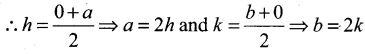Putting the values of a and b is eq (ii) and (iii) we get P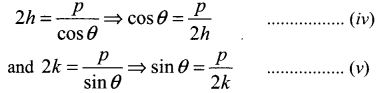Squaring and adding eq (iv) and (v) we get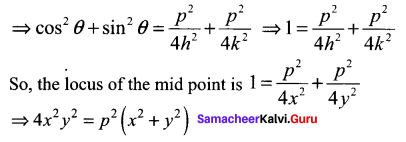Question 4.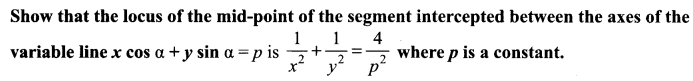Solution: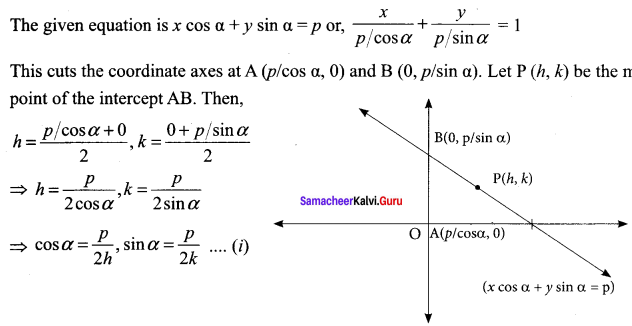Here, α is a variable. To find the locus of P (h, k), we have to eliminate α.
From (i), we obtain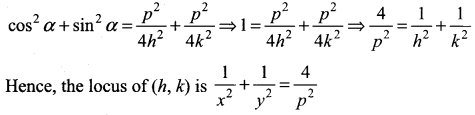Question 5.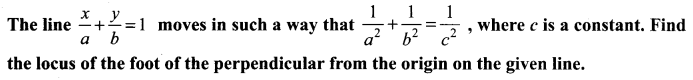Solution: